## Thursday, June 28, 2007

### Cool Papers at ICML 07

Here are a few that caught my eye:

• Scalable Training of L1-regularized Log-linear ModelsThe main idea is to do L-BFGS in an orthant where the gradient of the L1 loss doesn't change. Each time BFGS tries to step out of that orthant, project it's new point on the old orthant, and figure out the new orthant to explore

• Discriminative Learning for Differing Training and Test DistributionsIn addition to learning P(Y|X,t1), also learn P(this point is from test data|X). You can do logistic regression to model P(this point is from test data|X,t2), and then weight each point in training set by that value when learning P(Y|X). Alternatively you can learn both distributions simultaneously by maximizing P(Y|X,t1,t2) on test data, which gives even better results

• On One Method of Non-Diagonal Regularization in Sparse Bayesian Learning Relevance Vector Machine "fits" a diagonal Gaussian prior to data by maximizing P(data|prior).
In the paper they get a tractable method of fitting Laplace/Gaussian priors with non-diagonal matrices by first transforming parameters to a basis which uncorrelates the parameters at the point of maximum likelihood.

• Piecewise Pseudolikelihood for Efficient Training of Conditional Random FieldsDoing pseudo-likelihood training (replacing p(y1,y2|x) with p(y1|y2,x)p(y2|y1,x)) on small pieces of the graph (piece-wise training) gives better accuracy than pseudo-likelihood training on the true graph

• CarpeDiem: an Algorithm for the Fast Evaluation of SSL Classifiers -- a useful trick for doing Viterbi faster -- don't bother computing forward values for nodes which are certain to not be included in the best path. You know a node will not be included in the search path if the a+b+c is smaller than some other forward value on the same level. a+b+c is largest forward value on previous level, b is largest possible transition weight, c is the "emission" weight for that node

## Wednesday, June 27, 2007

### Machine Learning patents

I found a large number of machine learning related patent applications by doing a few queries on http://www.freepatentsonline.com/

Here are a couple that caught my eye:

• Logistic regression (A machine implemented system that facilitates maximizing probabilities)
• Boosting (A computer-implemented process for using feature selection to obtain a strong classifier from a combination of weak classifiers)
• Decision tree? (Machine learning by construction of a decision function)
• Bayesian Conditional Random Fields -- notably, Tom Minka is missing from the list of inventors

The interesting question is what the companies will do with those patents. The best thing they can do is to do nothing with them, which seems to be the case for most patents. The worst thing is they can go after regular users that make implementations of those methods freely available. For instance Xerox forced someone I know to remove a visualization applet from their webpage because the applet used hyperbolic space to visualize graphs, and they have patented this method of visualization. Here are some more worst-case scenarios

Does anyone have any other examples of notable machine learning patents/applications?

## Tuesday, June 12, 2007

### Log loss or hinge loss?

Suppose you want to predict binary y given x. You fit a conditional probability model to data and form a classifier by thresholding on 0.5. How should you fit that distribution?

Traditionally people do it by minimizing log-loss on data, which is equivalent to maximum likelihood estimation, but that has the property of recovering the conditional distribution exactly with enough data/modelling freedom. We don't care about exact probabilities, so in some sense it's doing too much work.

Additionally, log-loss minimization may sacrifice classification accuracy if it allows it to model probabilities better.

Here's an example, consider predicting binary y from real valued x. The 4 points give the possible 4 possible x values and their true probabilities. If you model p(y=1|x) as 1/(1+Exp(f_n(x))) where f_n is any n'th degree polynomial.

Take n=2, then minimizing log loss and thresholding on 1/2 produces Bayes-optimal classifier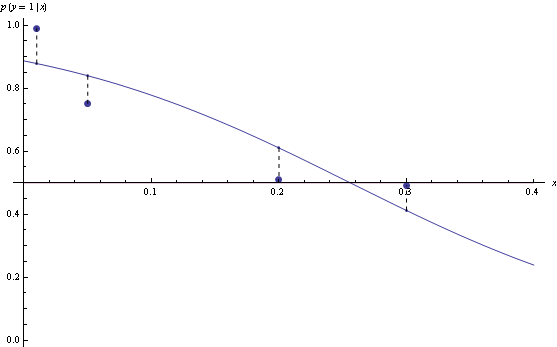However, for n=3, the model that minimizes log loss will have suboptimal decision rules for half the data.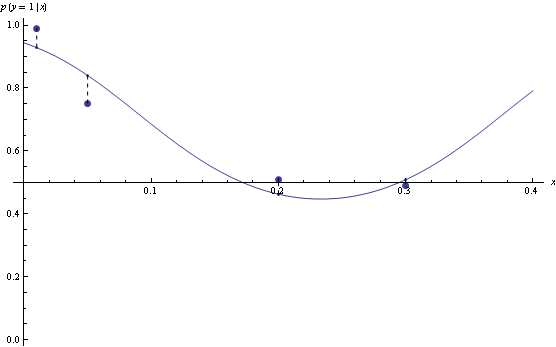Hinge loss is less sensitive to exact probabilities. In particular, minimizer of hinge loss over probability densities will be a function that returns returns 1 over the region where true p(y=1|x) is greater than 0.5, and 0 otherwise. If we are fitting functions of the form above, then once hinge-loss minimizer attains the minimum, adding extra degrees of freedom will never increase approximation error.

Here's example suggested by Olivier Bousquet, suppose your decision boundaries are simple, but the actual probabilities are complicated, how well will hinge loss vs log loss do? Consider the following conditional density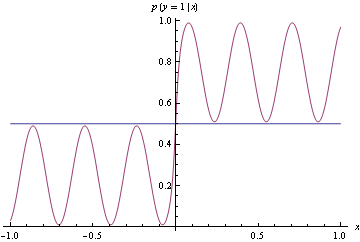Now use the conditional density functions of the same form as before, find minimizers of both log-loss and hinge loss. Hinge-loss minimization always produces Bayes optimal model for all n>1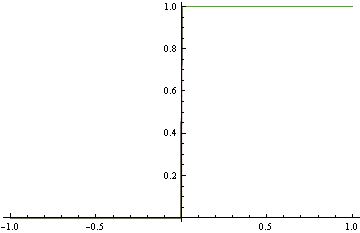When minimizing log-loss, the approximation error starts to increase as the fitter tries to match the exact oscillations in the true probability density function, and ends up overshooting.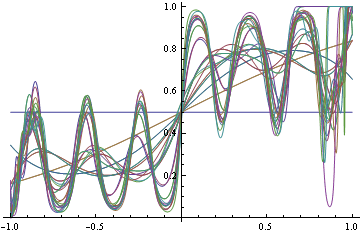Here's the plot of the area on which log loss minimizer produces suboptimal decision rule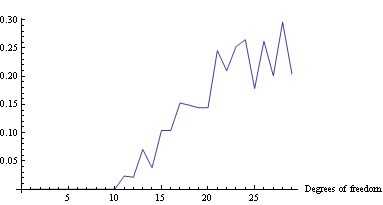Mathematica notebook (web version)